AtomicDensityMatrix`
AtomicDensityMatrix`

# Component

Component[tens,q]

extracts the qth component of a spherical tensor tens.

Component[cart,i]

extracts the ith component of a Cartesian vector cart.

Component[decomp, k]

extracts the spherical tensor of rank k from a tensor decomposition decomp.

Component[op,{m1,m2}]

extracts the matrix element corresponding to sublevels with angularmomentumprojection quantum numbers m1 and m2 from an operator op.

Component[sys,op,{s1,s2}]

extracts the matrix element of operator op corresponding to the states s1 and s2 of atomic system sys.

Component[sys,mat,{s1,s2}]

extracts the tensor decomposition from the tensordecomposition matrix mat corresponding to the states s1 and s2.

# Details and Options

• The component index q must be a integer or half-integer from the range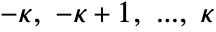, where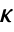is the rank of the spherical tensor.
• The quantum numbers mi be a integers or half-integers from the range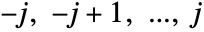, whereis the rank of the operator.
• The Cartesian component index i must be a positive integer.
• Additional indices or states ni may be given as Component[tens,n1,n2,] or Component[sys,tens,n1,n2,] to extract a component from a nested tensor structure. The index n1 refers to the top-level tensor, the index n2 to the second level, and so on.
• Component[tens] extracts the single component from a rankzero tensor, the single matrix element from a rank-zero operator, or the single tensor decomposition from a tensordecomposition matrix for a single angular-momentum state.

# Examples

open allclose all

## Basic Examples(1)

Thecomponent of a Cartesian vector:

The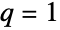component of a contravariant spherical tensor:

The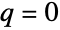component of a covariant tensor operator:

The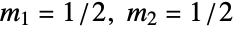matrix element of an operator on a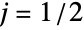angular-momentum space:

The rank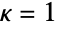tensor from a contravariant spherical-tensor decomposition:

## Scope (1)

The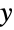component of a Cartesian vector operator:

The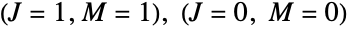matrix element of an operator:

The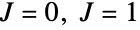tensor decomposition of a tensor decomposition matrix:

The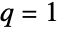component of the rank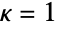spherical tensor of thetensor decomposition: